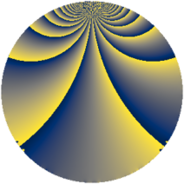# Properties

 Label 280.2.wLevel $280$ Weight $2$ Character orbit 280.w Rep. character $\chi_{280}(43,\cdot)$ Character field $\Q(\zeta_{4})$ Dimension $72$ Newform subspaces $1$ Sturm bound $96$ Trace bound $0$

# Learn more

## Defining parameters

 Level: $$N$$ $$=$$ $$280 = 2^{3} \cdot 5 \cdot 7$$ Weight: $$k$$ $$=$$ $$2$$ Character orbit: $$[\chi]$$ $$=$$ 280.w (of order $$4$$ and degree $$2$$) Character conductor: $$\operatorname{cond}(\chi)$$ $$=$$ $$40$$ Character field: $$\Q(i)$$ Newform subspaces: $$1$$ Sturm bound: $$96$$ Trace bound: $$0$$

## Dimensions

The following table gives the dimensions of various subspaces of $$M_{2}(280, [\chi])$$.

Total New Old
Modular forms 104 72 32
Cusp forms 88 72 16
Eisenstein series 16 0 16

## Trace form

 $$72q + 8q^{6} + O(q^{10})$$ $$72q + 8q^{6} + 16q^{10} - 16q^{12} - 8q^{16} + 8q^{17} - 28q^{18} - 20q^{20} - 36q^{22} - 8q^{25} - 32q^{26} - 4q^{30} + 40q^{32} + 64q^{36} - 4q^{40} + 20q^{42} - 64q^{43} + 48q^{46} - 80q^{48} - 32q^{51} + 16q^{52} - 24q^{56} + 4q^{58} - 80q^{60} - 40q^{62} - 8q^{65} + 32q^{66} - 24q^{68} + 40q^{72} - 40q^{73} + 112q^{75} - 8q^{76} + 28q^{78} - 20q^{80} - 72q^{81} + 24q^{82} + 80q^{83} + 8q^{86} - 88q^{88} + 136q^{90} - 96q^{92} - 56q^{96} - 8q^{97} + O(q^{100})$$

## Decomposition of $$S_{2}^{\mathrm{new}}(280, [\chi])$$ into newform subspaces

Label Dim. $$A$$ Field CM Traces $q$-expansion
$$a_2$$ $$a_3$$ $$a_5$$ $$a_7$$
280.2.w.a $$72$$ $$2.236$$ None $$0$$ $$0$$ $$0$$ $$0$$

## Decomposition of $$S_{2}^{\mathrm{old}}(280, [\chi])$$ into lower level spaces

$$S_{2}^{\mathrm{old}}(280, [\chi]) \cong$$ $$S_{2}^{\mathrm{new}}(40, [\chi])$$$$^{\oplus 2}$$